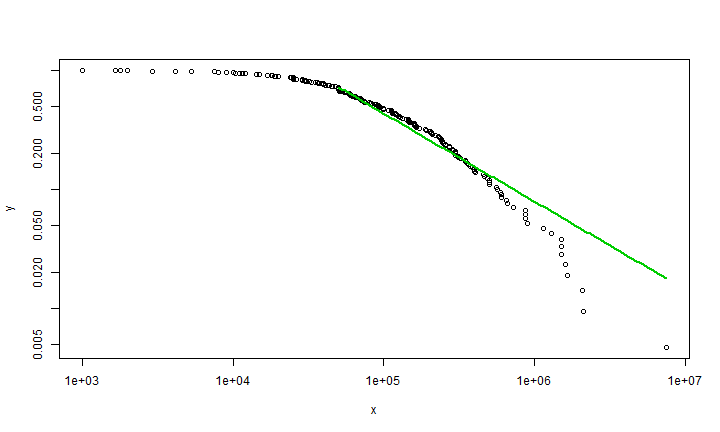# Power Law in R example

In this document I just want to show how to plot the data which following power law distribution.

``````library("poweRlaw")
``````
``````## Warning: package 'poweRlaw' was built under R version 3.1.1
``````

``````#knitr doesnt support for read.table using direct url
#if you want to get this data you can accessit in my github, under rischanlab.github.io project
setwd("C:/Users/rischan/Dropbox/RESEARCH/rischanlab.github.io")
``````

Show example data

``````head(blackouts)
``````
``````##       V1
## 1 570000
## 2 210882
## 3 190000
## 4  46000
## 5  17000
## 6 360000
``````

Run Power Law function

``````#Continuous power law objects take vectors as inputs,
m_bl = conpl\$new(blackouts\$V1)
#estimate the lower-bound
est = estimate_xmin(m_bl)
#update the distribution object
m_bl\$setXmin(est)
``````

Plot the data which following power law distribution

``````plot(m_bl)
lines(m_bl, col=2, lwd=2)
lines(m_bl, col=3, lwd=2)
``````